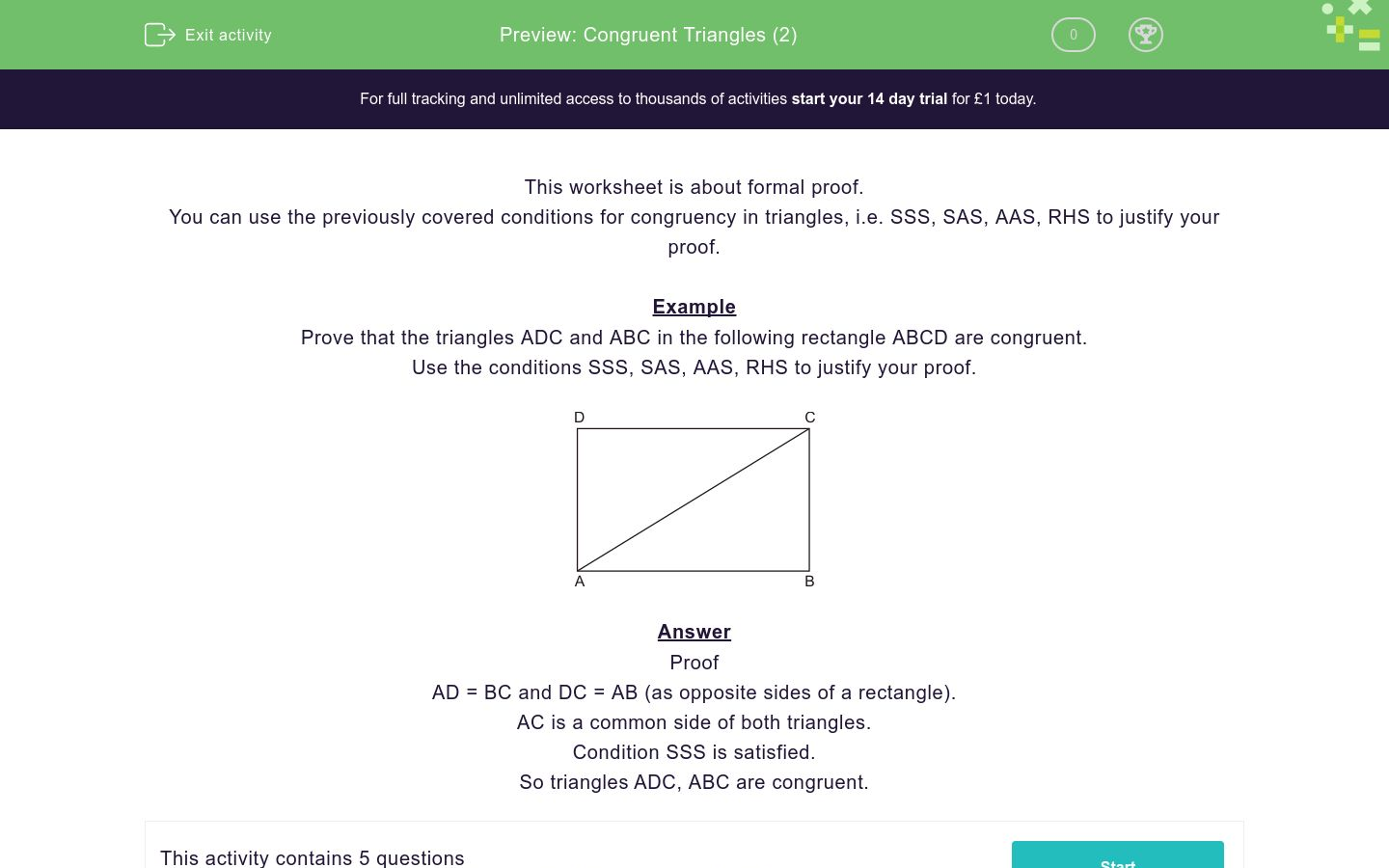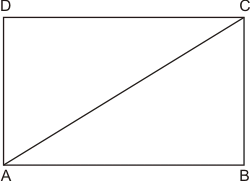# Congruent Triangles (2)

In this worksheet, students must formally prove that two triangles are congruent.Key stage:  KS 4

Curriculum topic:  Geometry and Measures

Curriculum subtopic:  Apply the Concepts of Congruence and Similarity

Difficulty level:### QUESTION 1 of 10

This worksheet is about formal proof.

You can use the previously covered conditions for congruency in triangles, i.e. SSS, SAS, AAS, RHS to justify your proof.

Example

Prove that the triangles ADC and ABC in the following rectangle ABCD are congruent.

Use the conditions SSS, SAS, AAS, RHS to justify your proof.Answer

Proof

AD = BC and DC = AB (as opposite sides of a rectangle).

AC is a common side of both triangles.

Condition SSS is satisfied.

So triangles ADC, ABC are congruent.

Prove that triangles WXY and WZY are congruent.

Use the conditions SSS, SAS, AAS, RHS to justify your proof.

WXYZ is a kite.Prove that triangles LKN and LMN are congruent.

Use the conditions SSS, SAS, AAS, RHS to justify your proof.

KLMN is a parallelogram.Prove that triangles ABC and ADC are congruent.

Use the conditions SSS, SAS, AAS, RHS to justify your proof.

ABCD is a rectangle.Prove that triangles PQT and SRT are congruent.

Use the conditions SSS, SAS, ASA, RHS to justify your proof.

PQ and RS are equal and parallel.Prove that triangles ABM and ACM are congruent.

Use the conditions SSS, SAS, ASA, RHS to justify your proof.

AM is perpendicular to BC. Triangle ABC is isosceles.• Question 1

Prove that triangles WXY and WZY are congruent.

Use the conditions SSS, SAS, AAS, RHS to justify your proof.

WXYZ is a kite.CORRECT ANSWER
EDDIE SAYS

Proof:

WY is a common side of both triangles.
WY is a bisector of angle XWZ (as a diagonal of a kite), so angles XWY and ZWY are equal.
Similarly, angles XYW and ZYW are equal too.
Condition ASA is satisfied, so Triangles WXY and WZY are congruent.
• Question 2

Prove that triangles LKN and LMN are congruent.

Use the conditions SSS, SAS, AAS, RHS to justify your proof.

KLMN is a parallelogram.CORRECT ANSWER
EDDIE SAYS

Proof:

KN = LM, NM = KL (as opposite sides of a parallelogram).
LN is a common side of both triangles.
Condition SSS is satisfied, so triangles LKN are LMN are congruent.
• Question 3

Prove that triangles ABC and ADC are congruent.

Use the conditions SSS, SAS, AAS, RHS to justify your proof.

ABCD is a rectangle.CORRECT ANSWER
EDDIE SAYS

Proof:

AD = BC, DC = AB (as opposite sides of a rectangle).
AC is a common side of both triangles.
Condition SSS is satisfied, so triangles ABC and ADC are congruent.

• Question 4

Prove that triangles PQT and SRT are congruent.

Use the conditions SSS, SAS, ASA, RHS to justify your proof.

PQ and RS are equal and parallel.CORRECT ANSWER
EDDIE SAYS

Proof:

Given PQ = RS.
Angles PQT and SRT are equal (alternate angles with parallel lines PQ and RS).
Similarly, angles QPT and RST are equal too.
Condition ASA is satisfied, so triangles PQT and SRT are congruent.
• Question 5

Prove that triangles ABM and ACM are congruent.

Use the conditions SSS, SAS, ASA, RHS to justify your proof.

AM is perpendicular to BC. Triangle ABC is isosceles.CORRECT ANSWER
EDDIE SAYS

Proof:

AB = AC.
AB is the hypotenuse of triangle ABM, AC is the hypotenuse of triangle ACM.
AM is the common side of both triangles.
Condition RHS is satisfied, so triangles ABM and ACM are congruent.
Preview ---- OR ----

Sign up for a £1 trial so you can track and measure your child's progress on this activity.

### What is EdPlace?

We're your National Curriculum aligned online education content provider helping each child succeed in English, maths and science from year 1 to GCSE. With an EdPlace account you’ll be able to track and measure progress, helping each child achieve their best. We build confidence and attainment by personalising each child’s learning at a level that suits them.

Get started#### Similar activities you may like

Start your £1 trial today.
Subscribe from £10/month.